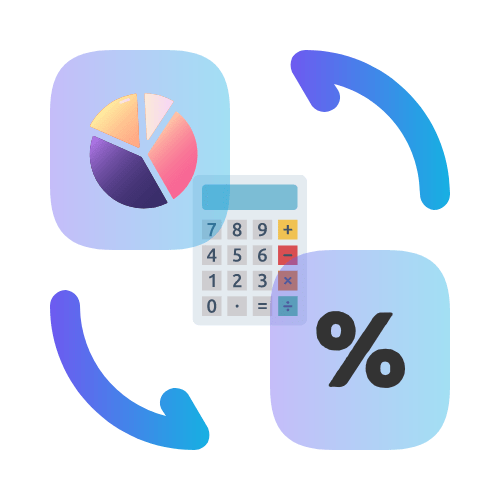# Fraction to Percent Calculator

## Convert fractions to percentages in seconds.

• Created by John Harris
• Reviewed by Ramesh Agarwal

The result is:

## Why Convert Fractions to Percentages?

Percentages provide a more visual approach to representing numbers. Compared to fractions, they are easier to understand and provide more flexibility when used. Percentages are a great way to compare numbers, measure progress and more.

## Are fractions and percentages the same?

No, fractions and percentages are not the same. In appearance, fractions take the form of 2/3, 4/5, 9/10 etc. Percentages are simply a way of expressing a fraction as a number out of one hundred. So 53% is the same as saying 53/100. If you have a fraction which is less than one, then you can express this as a percentage by using the word "percent". So 1/5 can be expressed as 20%.

## Where are fractions used in the real world?

Fractions are often used in cooking. For example, if you want to bake a cake, you will use ingredients like one cup of sugar, half a cup of oil, and three quarters of a cup of flour. You should also use fractions when building things.

For example, if you want to build a house, you will use one plank of wood, two planks of wood, and three planks of wood. Fractions are also used in areas like math and science. For example, you might need to measure a quarter of a metre.

## Why are fractions important?

Fractions are important in many aspects of everyday life. In school, fraction skills are crucial because they form the basis for lower math, such as decimals and percents. Fractions are used frequently in cooking and in recipes as they are an efficient way to represent quantities.

Fractions are commonly used as a measurement in business and finance. Without them we would not be able to express numbers in comparison with each other, making it almost impossible to measure stock quantities, ledger balances or staff productivity.

## What is the fraction to percent formula?

Percent is derived from the Latin word per centum, which means per hundred. Fraction to percentage consists of two parts: (i) numerator and (ii) denominator. Numerator is the top number, and denominator is the bottom number. Fractions can be turned into percentages in several different ways.

One approach is to multiply the numerator by 100, then divide by the denominator to get the final percentage. For example: 2/5 would be calculated as (2 x 100) = 200, then 200 / 5 = 40%.

An easier option is to use a fraction to percent calculator, like the one listed on this page. Fraction to percent calculators make it easy to calculate percentage from fractions as you just have to enter the fraction in it's current form and click the calculate button.

## Why is it helpful to write a fraction as a percent?

Fractions can be confusing when you're working with them in math. You can't just add them like decimals. To make working with fractions easier, it's helpful to convert the fraction to a percent (you can also convert percentages into fractions). That way you can do math with like decimals.

After converting the fraction to a percentage, then you can add like decimals and the answer will be a whole number.

## How are fractions and percentages different?

In a fraction, you are talking about a specific number out of a total; as in 2/5. In a percentage, you are talking about a number out of a hundred; as in 2%. Although both fractions and percentages share similarities, they are not the same.

Fractions are used to express division, and percentages are used to express a specific number or ratio in relation to another.

## FAQS

To convert a fraction to a percent, first convert it to a decimal by dividing the numerator by the denominator. Then, multiply the decimal by 100 to get the percentage value.

Certainly! For example, to convert the fraction 3/4 to a percent, first divide 3 by 4 to get 0.75. Then, multiply 0.75 by 100 to get 75%.

The fraction equivalent of 25% is 1/4. To find this, first convert 25% to a decimal (0.25), then express it as a fraction by using the decimal as the numerator and 100 as the denominator (0.25/1 = 25/100, which simplifies to 1/4).

Yes, you can also multiply the fraction by 100 and simplify if possible. For example, 3/8 becomes 37.5% when multiplied by 100.

To express a decimal as a percentage, multiply it by 100. For example, 0.6 becomes 60% when converted to a percentage.